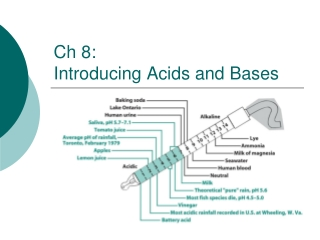DownloadDownload PresentationCh 8: Introducing Acids and Bases

# Ch 8: Introducing Acids and Bases

Télécharger la présentation## Ch 8: Introducing Acids and Bases

- - - - - - - - - - - - - - - - - - - - - - - - - - - E N D - - - - - - - - - - - - - - - - - - - - - - - - - - -
##### Presentation Transcript

1. Ch 8:Introducing Acids and Bases

2. pH of precipitation in the United States 2001, and in Europe as reported in 2002.

3. What are Acids and Bases? acid = substance that increases the concentration of H3O+ base = decreases the concentration of H3O+ (by increasing the amount of OH-)

4. Bronsted-Lowry Acid-Base Theory acid = proton donor (H+) base = proton acceptor HCl + H2O → HCl + NH3→

5. Conjugate Acid-Base Pairs

6. Relation Between [H+], [OH-], and pH H2O + H2O H3O+ + OH- H2O H+ + OH- Kw = [H+][OH-] = 1.01 x 10-14 at 25 oC equivalent

7. Example, p. 169: Concentration of H+ and OH- in Pure Water at 25 oC Calculate the concentrations of H+ and OH- in pure water at 25 oC.

8. As the concentration of H+ increases, OH- must decrease and vica-versa Example, p. 169: Finding [OH-] when H+ is Known. What is the concentration of OH- if [H+] = 1.0 x 10-3 M at 25 oC?

9. pH - a measure of the aciity of a solution ("puissance d'hydrogen") pH = -log [H+] (approximately!) [H+] = 10-3 M [H+] = 10.0 M [H+] = 10-10 M

10. Strengths of Acids and Bases strong =complete (100%) dissociation MEMORIZE these strong acids and bases - all other acids and bases are weak

11. HA H+ + A- OR HA + H2O H3O+ + A- B + H2O BH+ + OH- weak = incomplete dissociation

12. carboxylic acids = weak acids amines = weak bases RNH2 primary R2NH secondary R3N tertiary : : : Classes of Weak Acids and Bases polyprotic acids and bases H2CO3 CO32- H3PO4 PO43- Ca(OH)2

13. A- + H2O HA + OH- Relation Between Ka and Kb HA + H2O H3O+ + A- salt = conjugate baseundergoes hydrolysis

14. Example, p. 174: Ka for acetic acid is1.75 x 10-5. Find Kb for the acetate ion.

15. pH of solutions of strong acids and bases HA → H+ + A- BOH → B+ + OH- H2O H+ + OH- [H+] = [OH-] = 1.0 x 10-7 strong acids and bases completely dissociate Case I: concentration of acid or base >> 10-7

16. pH of a strong acid: Example p. 175 Find the pH of 4.2 x 10-3 M HClO4

17. Case II: concentration of acid or base  10-7 Now the contribution of H+ from water must be included -

18. pH of a strong base at a low concentration: “trick question” top of p. 176 Find the pH of 4.2 x 10-9 M KOH

19. pH of solutions of weak acids and bases (Sec 8-6, 8-7) – the “ICE” table Calculate the pH of a 0.020 M benzoic acid solution. Ka = 6.28 x 10-5 I: Exact solution using quadratic equation

20. II. Approximate solution

21. Weak Base Equilibrium, Example p. 183 Find the pH of a 0.0372 M solution of the commonly encountered (?) weak base cocaine. Kb = 2.6 x 10-6

22. Ch 9:Buffers buffer = resists changes in pH; solution of a weak acid or base and their salts

23. Henderson-Hasselbach Equation derivation and assumptions:

24. Example, p.191: Using the H-H Equation Sodium hypochlorite (NaOCl) was dissolved in a solution buffered to pH = 6.20. Find the ratio [OCl-]/[HOCl]

25. Example, p. 192: A Buffer Solution Find the pH of a solution prepared by dissolving 12.43 g of TRIS (FM = 121.136) plus 4.67 g TRIS hydrochloride (FM = 157.597) in 1.00 L of water.

26. How to Prepare a Buffer Solution • Consult a table of pKa's and pick the weak acid or base closest to the pH you need. • Solve for the ratio mol salt/(mol acid/base) • Choose a reasonable value for either mol salt or mol acid/base and solve for the other • After preparing the buffer, adjust the pH to the desired value (you never get exactly what you calculate because of the assumptions made in deriving the H-H equation

27. Example: buffer with pH = 4.8

28. Buffer Capacity: How well a solution resists changes in pH when an acid or base is added: when the pH = pKa!

29. Example, p.198 HA = H+ + A- mol A- = 0.0383, mol HA = 0.9617